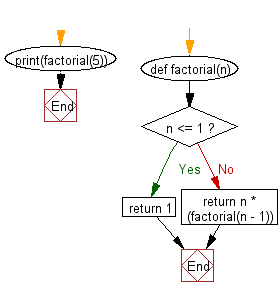﻿ Python Data Structures and Algorithms - Recursion: Factorial of a non-negative integer - w3resource# Python Data Structures and Algorithms - Recursion: Factorial of a non-negative integer

## Python Recursion: Exercise-4 with Solution

Write a Python program to get the factorial of a non-negative integer.

Sample Solution:

Python Code:

``````def factorial(n):
if n <= 1:
return 1
else:
return n * (factorial(n - 1))

print(factorial(5))
```
```

Sample Output:

```120
```

Flowchart:## Visualize Python code execution:

The following tool visualize what the computer is doing step-by-step as it executes the said program:

Python Code Editor:

What is the difficulty level of this exercise?

Test your Programming skills with w3resource's quiz.

﻿

## Python: Tips of the Day

Decapitalizes the first letter of a string:

Example:

```def tips_decapitalize(s, upper_rest=False):
return s[:1].lower() + (s[1:].upper() if upper_rest else s[1:])
print(tips_decapitalize('PythonTips'))
print(tips_decapitalize('PythonTips', True))
```

Output:

```pythonTips
pYTHONTIPS
```